### SOLUTIONS TO DIFFERENTIATION OF INVERSE TRIGONOMETRIC FUNCTIONS

SOLUTION 11 : Differentiatearc. What conclusion can be drawn from your answer about function y ? What conclusion can be drawn about functions arc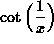and? First, differentiate, applying the chain rule to the inverse cotangent function. Then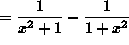= 0 .

If y' = 0 for all admissable values of x , then y must be a constant function, i.e.,for all admissable values of x ,

i.e.,

arcfor all admissable values of x .

In particular, if x = 1 , then

arc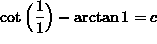i.e.,.

Thus, c = 0 and arcfor all admissable values of x . We conclude that

arc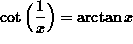.

Note that this final conclusion follows even more simply and directly from the definitions of these two inverse trigonometric functions.

SOLUTION 12 : Differentiate. Begin by applying the product rule to the first summand and the chain rule to the second summand. Then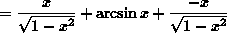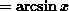.

SOLUTION 13 : Find an equation of the line tangent to the graph ofat x=2 . If x = 2 , then, so that the line passes through the point. The slope of the tangent line follows from the derivative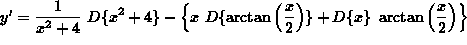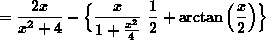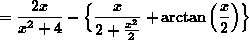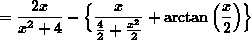(Recall that when dividing by a fraction, one must invert and multiply by the reciprocal. That is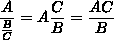.)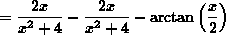.

The slope of the line tangent to the graph at x = 2 is.

Thus, an equation of the tangent line isoror.

SOLUTION 14 : Evaluate. Sinceand, it follows thattakes the indeterminate form `` zero over zero.'' Thus, we can apply L'H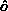pital's Rule. Begin by differentiating the numerator and denominator separately. DO NOT apply the quotient rule ! Then==(Recall that when dividing by a fraction, one must invert and multiply by the reciprocal. That is.)

=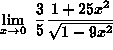==.

SOLUTION 15 : A movie screen on the front wall in your classroom is 16 feet high and positioned 9 feet above your eye-level. How far away from the front of the room should you sit in order to have the ``best" view ? Begin by introducing variables x and. (See the diagram below.)From trigonometry it follows that,

so that.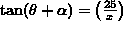so that.

It follows that,

that is, angleis explicitly represented as a function of distance x . Now find the value of x which maximizes the value of function. Begin by differentiating functionand setting the derivative equal to zero. Then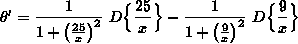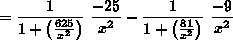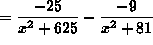.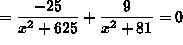.

Now solve this equation for x . Then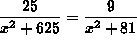iffiff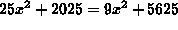iffiff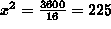ifffeet .

(Use the first or second derivative test (The first derivative test is easier.) to verify that this value of x determines a maximum value for.)

Thus, the ``best'' view is found x=15 feet from the front of the room.